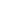Flat 50% off

Ends in

###### Applications of PMI for CBSE 11th Mathematics
860 views###### Applications of PMI for CBSE 11th Mathematics

Published on 19, Aug 2019

Theory and Problem Solving of Applications of PMI from Chapter Mathematical Induction for class 11th CBSE Mathematics at Misostudy. This lecture is in English. The Principle of Mathematical Induction (PMI) is a method for proving statements. “Mathematical induction” is something totally different. It refers to a kind of deductive argument, a logically rigorous method of proof. It works because of how the natural numbers are constructed from set theory. Mathematical induction can be used to prove that the following statement, P(n), holds for all natural numbers n. P(n) gives a formula for the sum of the natural numbers less than or equal to number n. Watch full video to know more. Crack CBSE Mathematics Get Important theory and exam pattern question exercise for CBSE preparation with Misostudy's expert faculties. Get started for your CBSE 2020 Preparation with Misostudy. Misostudy's courses will help students to boost for your CBSE preparation to perform best in exams and achieve a good rank.